Courses

# Introduction to Kinematics and Relative Velocity Class 11 Notes | EduRev

## Class 11 : Introduction to Kinematics and Relative Velocity Class 11 Notes | EduRev

The document Introduction to Kinematics and Relative Velocity Class 11 Notes | EduRev is a part of the Class 11 Course Physics Class 11.
All you need of Class 11 at this link: Class 11

Kinematics is the science of motion. In human movement, it is the study of the positions, angles, velocities, and accelerations of body segments and joints during motion.
It's important to define these terms precisely.
Body segments are considered to be rigid bodies for the purposes of describing the motion of the body. They include the foot, shank (leg), thigh, pelvis, thorax, hand, forearm, upper-arm and head.
Joints between adjacent segments include the ankle (talocrural plus subtalar joints), knee, hip, wrist, elbow and shoulder.
Position describes the location of a body segment or joint in space, measured in metres. A related measure called displacement refers to the position with respect to a starting position. In two dimensions, the position is given in Cartesian co-ordinates, with horizontal followed by vertical position.
e.g.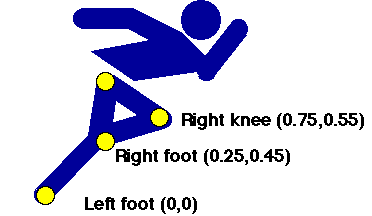In three dimensions all three directions must be defined:
e.g. (1.5, 6.2, 3.2), (2.8, 9.6, 7.8) etc.

Joint angle (also called inter-segmental angle) is the simply the angle between the two segments on either side of the joint, usually measured in degrees and often converted to clinical notation. Since joint angles are relative to the segment angles, they don't change with the body orientation.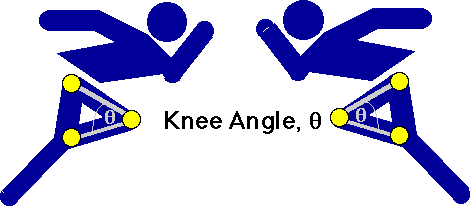Segment angle is quite different. It is the angle of the segment with respect to the right-hand horizontal. Note that it is an absolute measure, meaning that it changes according to the orientation of the body.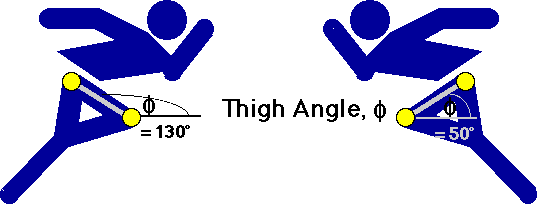Velocity is another word for speed. Velocity may be linear (change in position) measured in metres per second (m/s or ms-1), or angular (change in angle), measured in degrees per second (deg./s or deg.s-1). Normally, velocity is derived from position or angle data by the process of differentiation.
Velocity = distance moved / time taken

Thus if the knee moves from a horizontal position of 1.5 m to a position of 1.6 m in 1/50 of a second, it has a velocity of
Velocity = (1.6 - 1.5) / (1/50)
= 0.1 x 50 = 5 ms-1.

Acceleration is change in velocity. Again, it may be linear (change in linear velcocity) measured in metres per second per second (m/s2 or ms-2), or angular (change in angular velocity), measured in degrees per second per second (deg./s2 or deg.s-2). Acceleration, too, is usually calculated from the position data by differentiating twice. It can also be measured directly by an instrument called an accelerometer.

Relative Velocity
The velocity of an object only really has meaning when it is expressed relative to a set of axes or 'frame of reference'.

We usually consider the motion of objects moving relative to the Earth, but since the Earth is orbiting the Sun and the Sun is moving through the Galaxy this is a purely arbitrary concept. When dealing with more than one body in motion the problem is complex - consider the difficulties in sending the space probe Giotto to rendezvous with Halley's comet many millions of miles away!

We should always specify the frame of reference relative to which an object is moving.

The velocity of one body relative to another is called its relative velocity

Think about the case where both bodies are moving relative to a third. A simple example would be where the motions of both bodies are in the same straight line - for instance, two cars travelling along a motorway. If both cars are travelling in the same direction, one at 25 ms-1 and the other at 35 ms-1 then their relative velocity is 10 ms-1 (by vector addition).

If they are moving in opposite directions, however, the relative velocity of one car with respect to the other is therefore 60ms-1 (See Figure 1).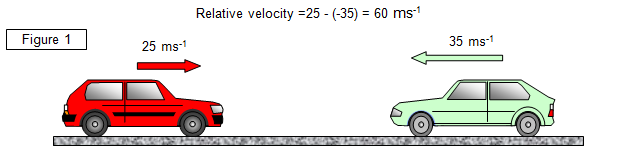What we are effectively doing is considering one car to be at rest and finding the velocity of the other car in that frame of reference. To do this we must add the negative of the velocity of one car to both cars' velocities. This effectively brings one to rest and we then consider the velocity of the other car relative to it.

The situation is a little more complex when the motion of the two objects is not in the same straight line. Consider the case shown in Figure 2.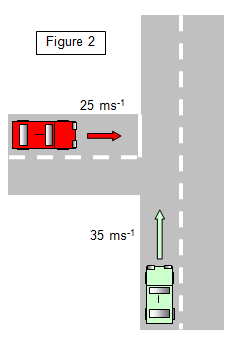Here the two cars are still moving at the same speeds but this time at right angles to each other as they approach a junction.

The relative velocity is found by using a vector triangle.

If we add the negative of the velocity of the red car (-25) to both the velocity of the red car and the green car we can imagine the red car to be at rest and then find the velocity of the green car relative to it. (See Figure 3).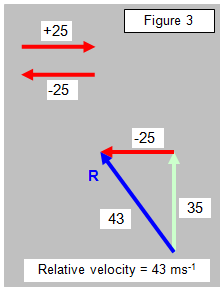This is most easily done by drawing the vector diagram. The resultant shown by the vector R is the velocity of the green car relative to the red car. In our example the velocity of the green car relative to the red car is 43 ms-1 and in a direction of 325o.
Think about the result and see if it matches your common sense view of how you would see the green car moving if you were in the red car. It would seem to be moving up the page and towards the left. It does actually fit the result!

Relative motion at angles other than 90o
We will consider the case where the cars are not moving at right angles to each other.

In fact we will not consider cars at all but the two aeroplanes shown in Figure 4 (a).

To find the velocity of plane A relative to plane B we must 'make plane B stationary'. We do this by adding the negative of plane B's velocity to both planes. If we were on plane B it would be like making that plane stop and we would then see plane A moving relative to us. This is done in Figure 4(b).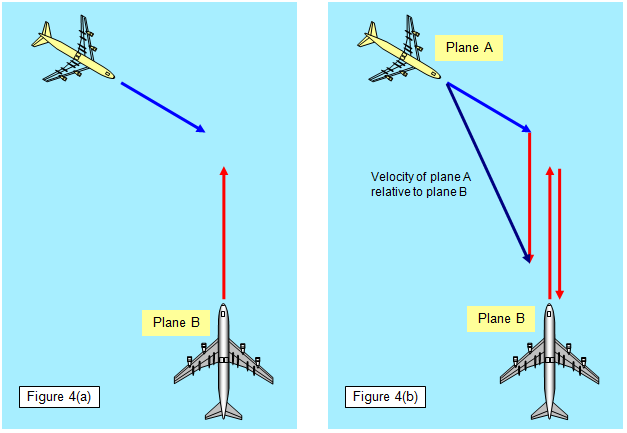Offer running on EduRev: Apply code STAYHOME200 to get INR 200 off on our premium plan EduRev Infinity!

,

,

,

,

,

,

,

,

,

,

,

,

,

,

,

,

,

,

,

,

,

;